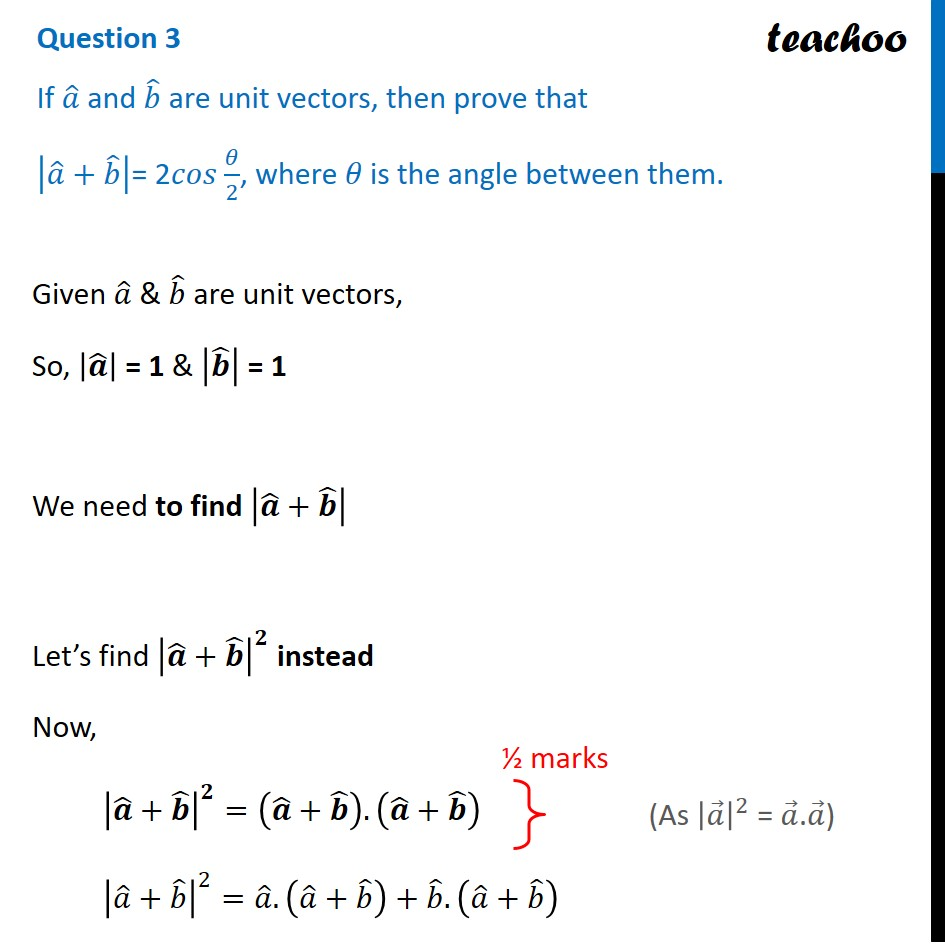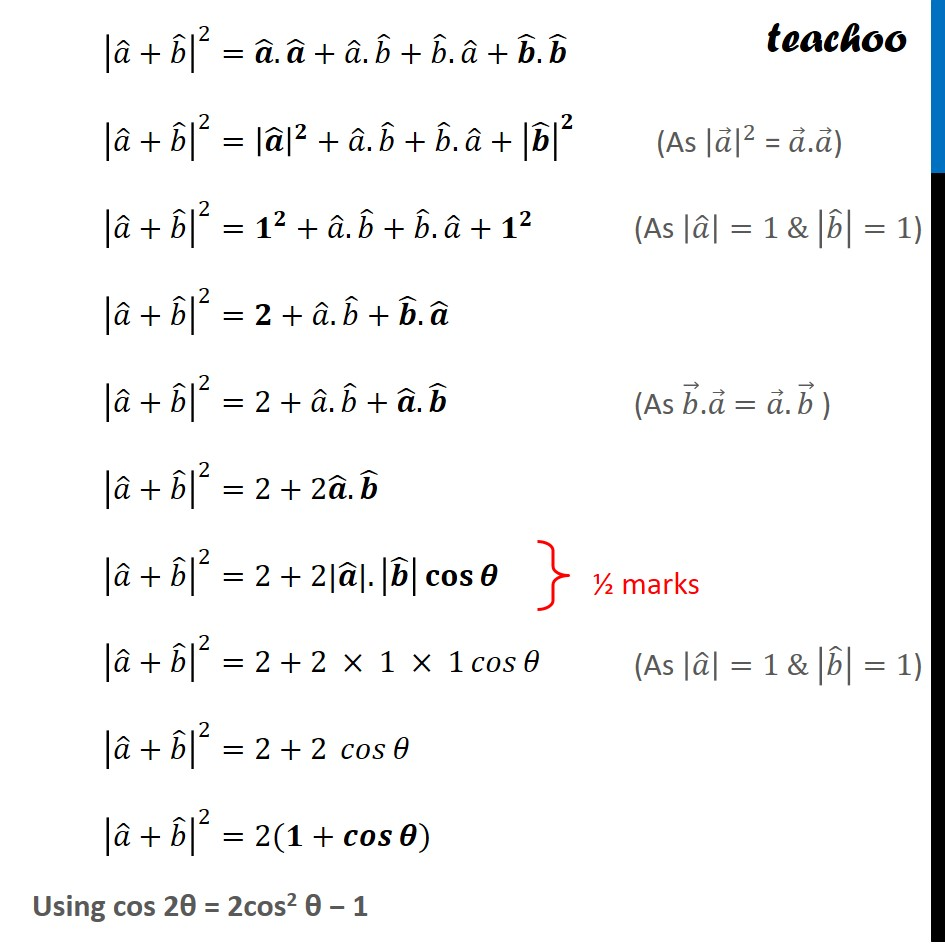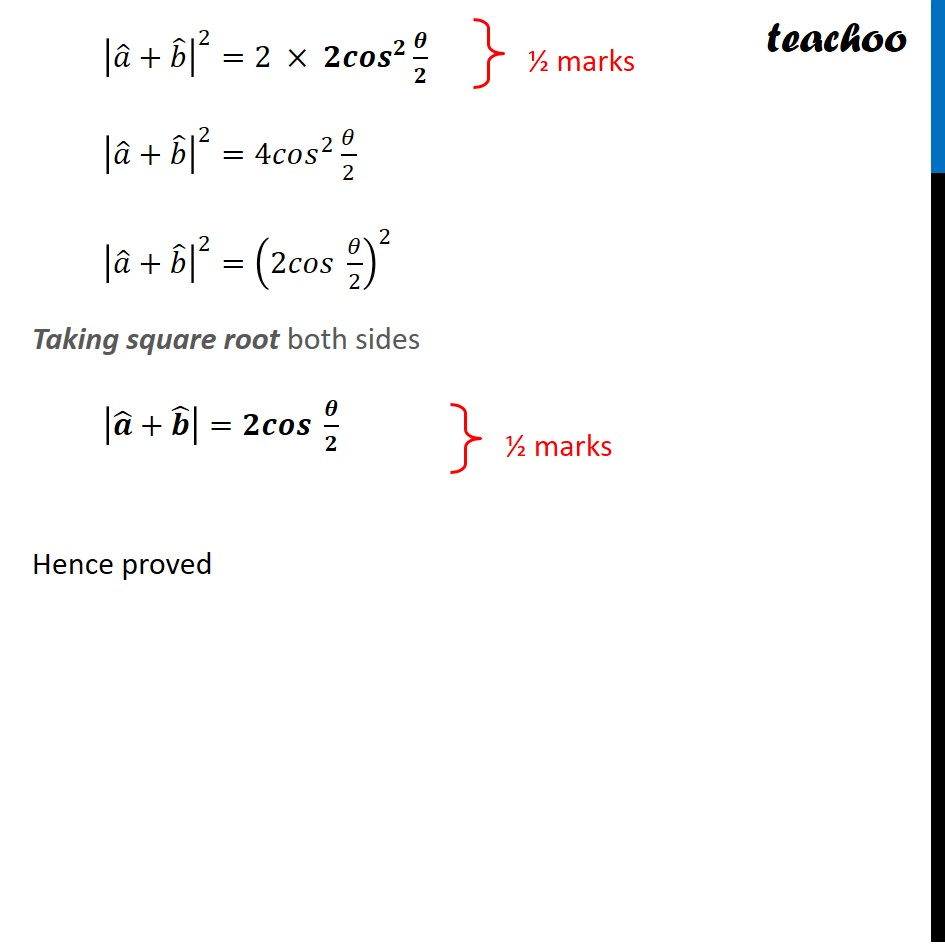CBSE Class 12 Sample Paper for 2022 Boards (For Term 2)

Class 12
Solutions of Sample Papers and Past Year Papers - for Class 12 Boards

## |a ̂+b ̂ |= 2𝑐𝑜𝑠 θ/2, where 𝜃 is the angle between them.

This question is similar to MIsc 17 (MCQ) - Chapter 10 Class 12 - Vector AlgebraLearn in your speed, with individual attention - Teachoo Maths 1-on-1 Class

### Transcript

Question 3 If 𝑎 ̂ and 𝑏 ̂ are unit vectors, then prove that |𝑎 ̂+𝑏 ̂ |= 2𝑐𝑜𝑠 𝜃/2, where 𝜃 is the angle between them. Given 𝑎 ̂ & 𝑏 ̂ are unit vectors, So, |𝒂 ̂ | = 1 & |𝒃 ̂ | = 1 We need to find |𝒂 ̂+𝒃 ̂ | Let’s find |𝒂 ̂+𝒃 ̂ |^𝟐 instead Now, |𝒂 ̂+𝒃 ̂ |^𝟐=(𝒂 ̂+𝒃 ̂ ).(𝒂 ̂+𝒃 ̂ ) |𝑎 ̂+𝑏 ̂ |^2=𝑎 ̂.(𝑎 ̂+𝑏 ̂ )+𝑏 ̂.(𝑎 ̂+𝑏 ̂ ) (As |𝑎 ⃗ |^2 = 𝑎 ⃗.𝑎 ⃗) |𝑎 ̂+𝑏 ̂ |^2=𝒂 ̂.𝒂 ̂+𝑎 ̂.𝑏 ̂+𝑏 ̂.𝑎 ̂+𝒃 ̂.𝒃 ̂ |𝑎 ̂+𝑏 ̂ |^2=|𝒂 ̂ |^𝟐+𝑎 ̂.𝑏 ̂+𝑏 ̂.𝑎 ̂+|𝒃 ̂ |^𝟐 |𝑎 ̂+𝑏 ̂ |^2=𝟏^𝟐+𝑎 ̂.𝑏 ̂+𝑏 ̂.𝑎 ̂+𝟏^𝟐 |𝑎 ̂+𝑏 ̂ |^2=𝟐+𝑎 ̂.𝑏 ̂+𝒃 ̂.𝒂 ̂ |𝑎 ̂+𝑏 ̂ |^2=2+𝑎 ̂.𝑏 ̂+𝒂 ̂.𝒃 ̂ |𝑎 ̂+𝑏 ̂ |^2=2+2𝒂 ̂.𝒃 ̂ |𝑎 ̂+𝑏 ̂ |^2=2+2|𝒂 ̂|.|𝒃 ̂ | 𝐜𝐨𝐬⁡𝜽 |𝑎 ̂+𝑏 ̂ |^2=2+2 × 1 × 1 𝑐𝑜𝑠⁡𝜃 |𝑎 ̂+𝑏 ̂ |^2=2+2 𝑐𝑜𝑠⁡𝜃 |𝑎 ̂+𝑏 ̂ |^2=2(𝟏+𝒄𝒐𝒔⁡𝜽) Using cos 2θ = 2cos2 θ − (As |𝑎 ⃗ |^2 = 𝑎 ⃗.𝑎 ⃗) (As |𝑎 ̂ |=1 & |𝑏 ̂ |=1) (As |𝑎 ̂ |=1 & |𝑏 ̂ |=1) |𝑎 ̂+𝑏 ̂ |^2=2 × 𝟐𝒄𝒐𝒔^𝟐 𝜽/𝟐 |𝑎 ̂+𝑏 ̂ |^2=4𝑐𝑜𝑠^2 𝜃/2 |𝑎 ̂+𝑏 ̂ |^2=(2𝑐𝑜𝑠 𝜃/2)^2 Taking square root both sides |𝒂 ̂+𝒃 ̂ |=𝟐𝒄𝒐𝒔 𝜽/𝟐 Hence proved